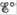# The solubility product for CuI(s) is 1.1 × 10 −2 Calculate the value of for the half-reaction CuI ( s ) + e − → Cu ( s ) + I − ( a q )### Chemistry: An Atoms First Approach

2nd Edition
Steven S. Zumdahl + 1 other
Publisher: Cengage Learning
ISBN: 9781305079243

#### Solutions

Chapter
Section### Chemistry: An Atoms First Approach

2nd Edition
Steven S. Zumdahl + 1 other
Publisher: Cengage Learning
ISBN: 9781305079243
Chapter 17, Problem 92E
Textbook Problem
11 views

## The solubility product for CuI(s) is 1.1 × 10−2 Calculate the value offor the half-reaction CuI ( s ) + e − → Cu ( s ) + I − ( a q )

Interpretation Introduction

Interpretation:

A half-cell reaction is given. The value of E° for the given cell reaction is to be calculated.

Concept introduction:

The relationship between reduction potential and standard reduction potential value and activities of species present in an electrochemical cell at a given temperature is given by the Nernst equation.

The value of Ecell is calculated using Nernst formula,

E=E°(RTnF)ln(Q)

At room temperature the above equation is specifies as,

E=E°(0.0591n)log(Q)

This relation is further used to determine the relation between ΔG° and K , ΔG° and E°cell .

Solubility product is applied only for those ionic compounds that are sparingly soluble. The product of solubility of ions is called solubility product and solubility is present in moles per liter.

To determine: The value of cell potential E° for the given cell reaction.

### Explanation of Solution

Given

The cell reaction is,

CuI(s)+eCu(s)+I(aq)

The value of standard reduction potential is,

Cu++eCuE°=0.52V

The value of solubility product constant is,

CuI(s)Cu+(aq)+I(aq)Ksp=1.1×1012

The reaction involves the transfer of 1 mole of electrons.

The standard cell potential at room temperature can be calculated by using the formula,

E°=0.0591nlogKsp

Where,

• Eo is the standard cell potential.
• n is the number of moles of electron transfer.
• Ksp is the solubility product constant.

Substitute the values of n and Ksp in the above equation,

E°=0.0591nlogKsp=0.05911log(1

### Still sussing out bartleby?

Check out a sample textbook solution.

See a sample solution

#### The Solution to Your Study Problems

Bartleby provides explanations to thousands of textbook problems written by our experts, many with advanced degrees!

Get Started

Find more solutions based on key concepts
Blood LDL values of people eating typical, meat-rich Western diets are generally higher than LDL values of vege...

Nutrition: Concepts and Controversies - Standalone book (MindTap Course List)

List the basic types of membranes in the body.

Human Biology (MindTap Course List)

The mayor of a city reprimands some city employees because they will not remove the obvious sags from the cable...

Physics for Scientists and Engineers, Technology Update (No access codes included)

Which body nominally governs the Law of the Sea?

Oceanography: An Invitation To Marine Science, Loose-leaf Versin